Next: Bibliography Up: E. Diffraction in Far Previous: E.2 Circular Aperture

# E.3 Rectangular Aperture

Figure E.6 shows the aperture plane with the coordinates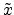and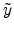.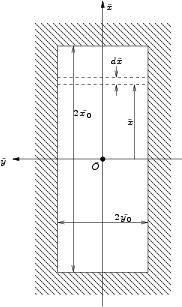The transmission function is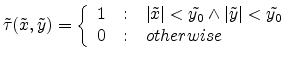(E.54)

The area of the aperture is given by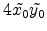. The FOURIER transform can be calculated from (E.27) and splitted into two integrals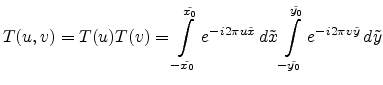(E.55)

One stripe in Figure E.6 is thereby given by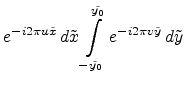(E.56)

Integration of (E.55) is simple and straightforward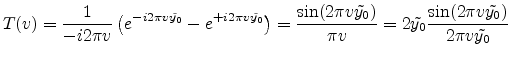(E.57)

The rightmost term can be defined as a new function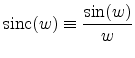(E.58)

With this substitution (E.57) yields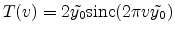(E.59)

and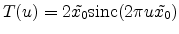(E.60)

in an analogous way. Therefore in Point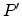the electric field is according to (E.29)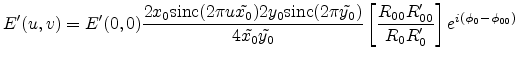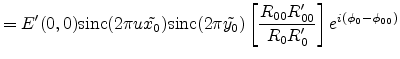(E.61)

and the intensity as the square of the electrical field is then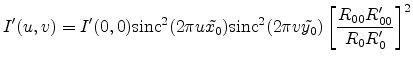(E.62)

For the special case of the source being located on the z-axis, the coordinates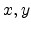are zero and the coordinates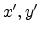are the following functions of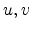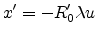and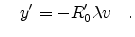(E.63)

For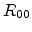and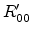(the direct beam) the coordinates are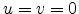and according to (E.63). The direct beam is therefore in the z-axis and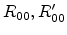can be written as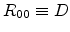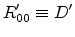(E.64)

Together with (E.14) and (E.64) the square of the fraction in (E.62) gives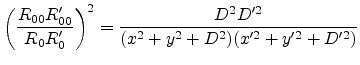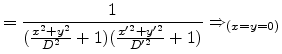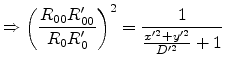(E.65)

Therefore this fraction can be set to unity if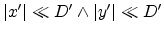. This assumption yields finally for the intensity behind a rectangular aperture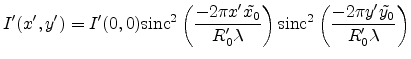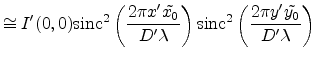(E.66)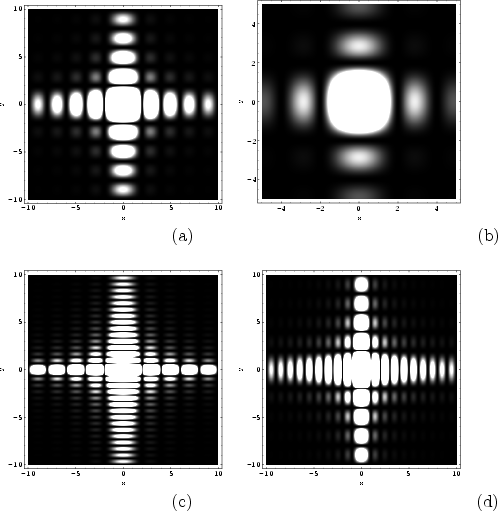Next: Bibliography Up: E. Diffraction in Far Previous: E.2 Circular Aperture

R. Minixhofer: Integrating Technology Simulation into the Semiconductor Manufacturing Environment